# Ensemble Learning: 5 Main Approaches

We outline the most popular Ensemble methods including bagging, boosting, stacking, and more.comments

By Diogo Menezes Borges, Data Scientist.

Remember a few months ago when everyone was taking wild guesses at the color of the dress (blue or gold) or the tennis shoe (pink or grey)? For me, Ensemble Learning looks a bit like that. A group of weak learners comes together to form a strong learner, thus increasing the accuracy of any Machine Learning model.

Have you ever heard about Wisdom of the Crowd? Not the TV Series, but the real term. No? Ok…so, imagine you ask a complex question to thousands of random people. Now, aggregate their answers.

What you will probably find is that the answer given by the majority is better than the expert’s answer.

The wisdom of the crowd is the collective opinion of a group of individuals rather than that of a single expertWikipedia

Returning to the field of Machine Learning, we can apply the same idea. For example, if we aggregate the predictions of a group of predictors (such as classifiers and regressors), we will probably get a better prediction than with the best individual predictor.

A group of predictors is called an ensemble. Therefore this Machine Learning technique is known as Ensemble Learning. Voilá!

In my last post “A breath of fresh air with Decision Trees”, I talked about the algorithm Decision Tree and how we can use it to make predictions. As you know, in Nature, a group a trees makes a forest. Hum… so that means..

Imagine that I train a group of Decision Trees classifiers to make individual predictions according to different subsets of the training dataset. Later I predict the expected class for an observation, taking into account what the majority predicted for the same instance. So… that means, I am basically using the knowledge of an ensemble of Decision Tree or as it is mostly known a Random Forest.

For this post we will go through the most popular Ensemble methods including bagging, boosting, stacking and a few others. Before we go into detail please have the following in mind:

“Ensemble methods work best when the predictors are as independent of one another as possible. One way to get diverse classifiers is to train them using very different algorithms. This increases the chance that they will make very different types of errors, improving the ensemble’s accuracy.”Hands-on Machine Learning with Scikit-Learn & TensorFlow”, Chapter 7

#### Simple Ensemble Techniques

• Hard Voting Classifier

This is the most simple example of the technique and we’ve already kindly approached it. Voting classifiers are usually used in classification problems. Imagine you trained and fitted a few classifiers (Logistic Regression classifier, SVM classifiers, Random Forest classifier, among others) to your training dataset.

A simple way to create a better classifier is to aggregate the prediction made by each one and set the majority chosen as the final prediction. Basically, we can consider this as if we’re retrieving the mode of all predictors.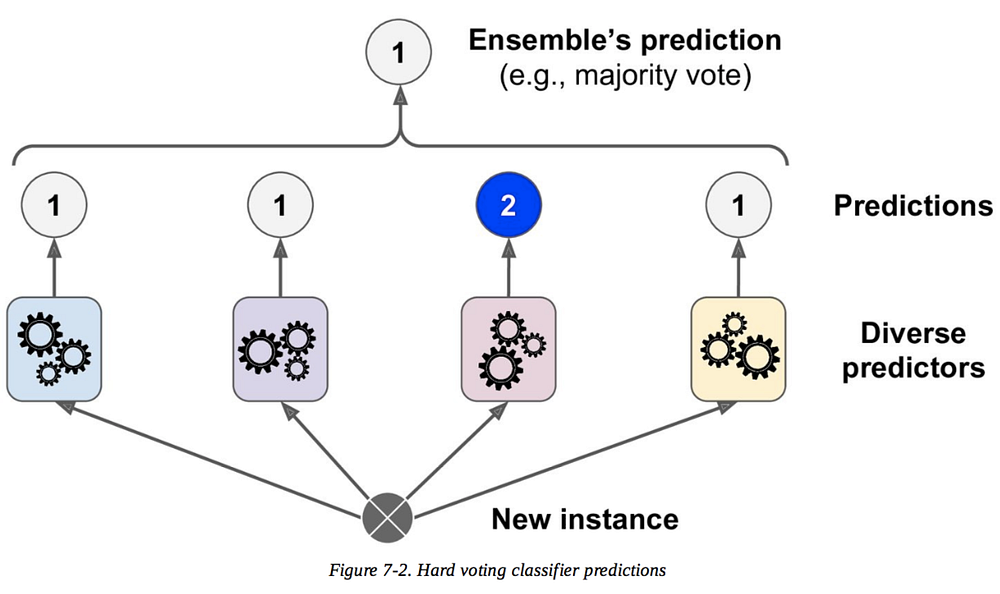“Hands-on Machine Learning with Scikit-Learn & TensorFlow”, Chapter 7

• Averaging

The first example we’ve seen is mostly used for classification problems. Now let’s take a look at a technique used for Regression problems known as Averaging. Similar to Hard Voting, we take multiple predictions made by different algorithms and take its average to make the final prediction.

• Weighted Averaging

This technique is equal to Averaging with the twist that all models are assigned different weights according to its importance to the final prediction.

• Stacking

Also known as Stacked Generalisation, this technique is based on the idea of training a model that would perform the usual aggregation we saw previously.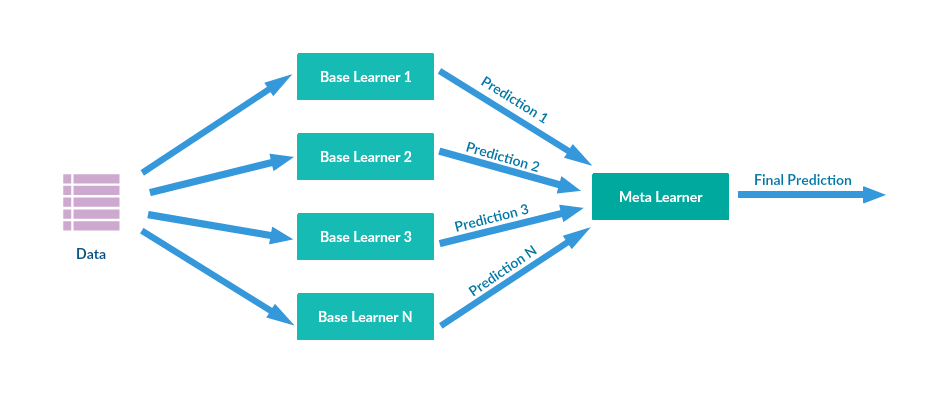We have N predictors, each making its predictions and returning a final value. Later, the Meta Learner or Blender will take these predictions as input and make the final prediction.

Let’s see how it works…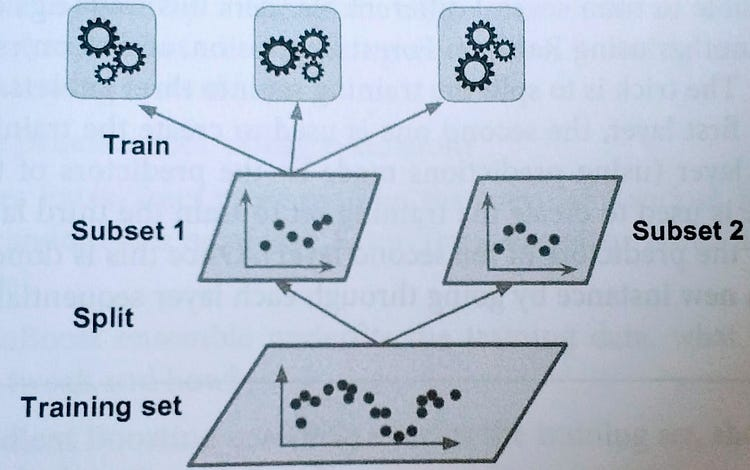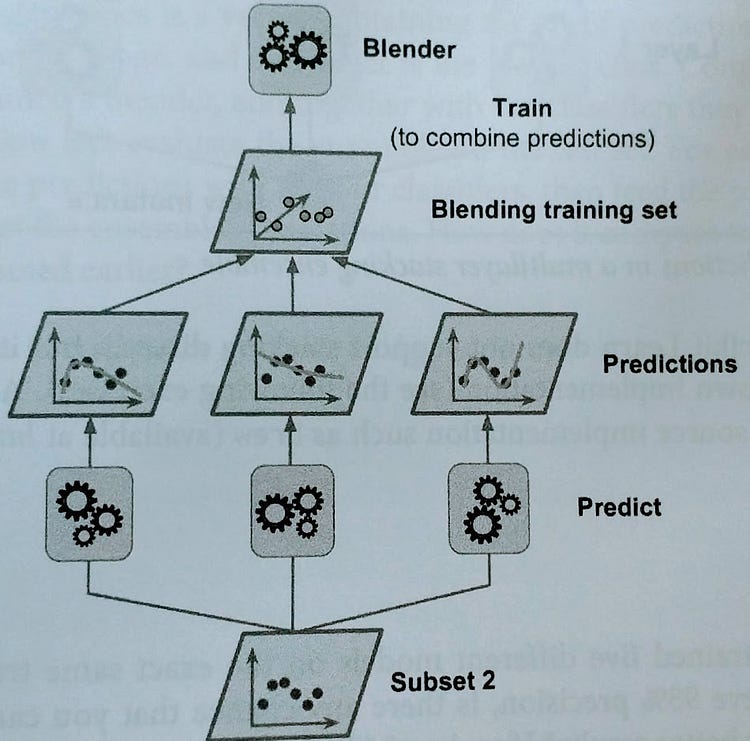The common approach to train the Meta Learner is a hold-out set. Firstly, you split the training set into two subsets. The first subset of data is used to train the predictors.

Later, the same predictors that trained on the first subset are used to make predictions on the second (held-out) set. By doing this, the cleanness of the predictions is ensured since those algorithms never saw the data.

With these new predictions, we are able to generate a new training set which will be used as input features (making the new training set three-dimensional) and keeping the target values.

Therefore, in the end, the Meta Learner is trained on this new dataset and it predicts the target values having into account the values given by the first predictions.

Moreover, you can train several Meta Learner using this methodology (e.g. one using Linear Regression, another one using Random Forest Regression, etc). To use more than one Meta Learner you have to split the training set into three or more subsets: the first to train the first layer of predictors, the second used to make predictions on unknown data and create a new dataset and the third one used to train the Meta Learner and make the predictions for the target values.

• Bagging and Pasting

Another approach is to use the same algorithm for every predictor (e.g. all decision tree), however, having different random subsets of the training set allowing for a more generalised result.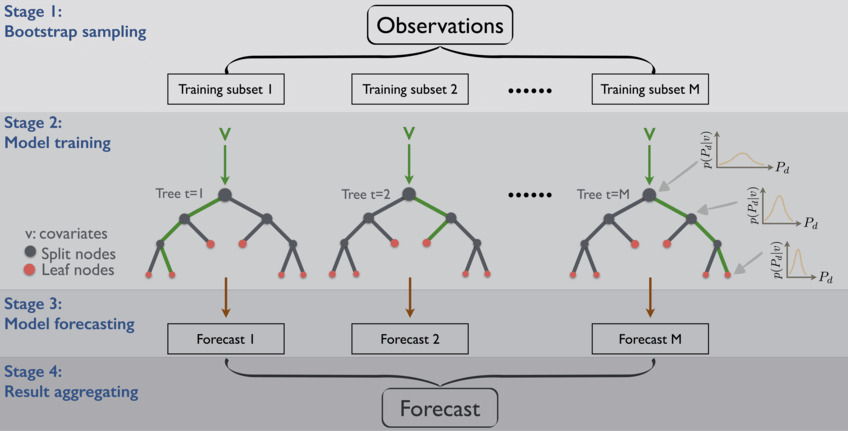Article “Spatial downscaling of precipitation using adaptable random forests

Regarding the creation of the subsets, you can either proceed with replacement or without. If we assume there is replacement, some observations maybe are present in more than one subset, the reason why we call this method bagging (short for bootstrap aggregating). When the sampling is performed without replacement we ensure that all the observations, in each subset, are unique thus guaranteeing there are no repeated observations in every subset.

Once all the predictors are trained, the ensemble can make a prediction for a new instance by aggregating the predicted values of all trained predictors, just like we saw in the hard voting classifier. Although each individual predictor has a higher bias than if it were trained on the original dataset, the aggregation allows the reduction of both bias and variance (check my post on the bias-variance trade-off)

With pasting, there is a high chance that these models will give the same result since they are getting the same input. Bootstraping introduces more diversity in each subset than pasting, therefore you can expect a higher bias from bagging which means the predictors are less correlated so the ensemble’s variance is reduced. Overall, the bagging model usually offers better results which explains why generally you hear more about bagging than pasting.

• Boosting

If a data point is incorrectly predicted by the first model as well as the next (probably all models), will the combination of their results provide a better prediction? This is where Boosting comes into action.

Also known as Hypothesis Boosting, it refers to any Ensemble method that can combine weak learners into a stronger one. It’s a sequential process where each subsequent model attempts to fix the errors of its predecessor.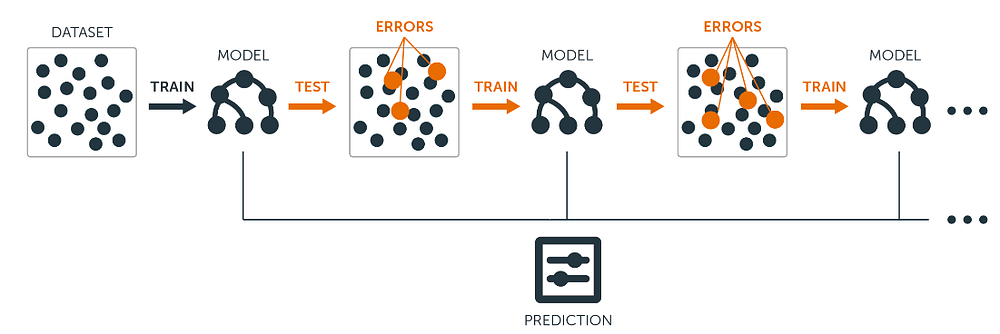Source

Each model would not perform well on the entire dataset. However, they do have great performances in parts of the dataset. Hence, from Boosting we can expect that each model will actually contribute to boosting the performance of the overall ensemble.

#### Algorithms based on Bagging and Boosting

The most commonly used Ensemble Learning techniques are Bagging and Boosting. Here are some of the most common algorithms for each of these techniques. I’ll write single posts presenting each one of these algorithms in the near future.

Bagging algorithms:

Boosting algorithms:

Bio: Diogo Menezes Borges is a Data Scientist with a background in engineering and 2 years of experience using predictive modeling, data processing, and data mining algorithms to solve challenging business problems.

Original. Reposted with permission.

Resources:

Related: